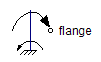Hyperbolic Cosine - MapleSim Help

Hyperbolic Cosine

Compute the hyperbolic cosineDescription The Hyperbolic Cosine (or Cosh) component computes the element-wise hyperbolic cosine. The Signal Size parameter assigns the dimension of the input and output connectors.
 Equations ${y}_{j}=\mathrm{cosh}\left({u}_{j}\right)$

Connections

 Name Description Modelica ID $u$ Real input vector u $y$ Real output vector y

Parameters

 Name Default Units Description Modelica ID Signal Size $1$ Dimension of input and output signals signalSize

 Modelica Standard Library The component described in this topic is from the Modelica Standard Library. To view the original documentation, which includes author and copyright information, click here.